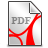# A global unique H(1/2) (potential energy) inner product based weak solution of the 3D-Navier-Stokes equations

We provide a global unique (weak, generalized Hopf) H(1/2)-solution of the generalized 3D Navier-Stokes initial value problem. The global boundedness of a generalized energy inequality with respect to the energy Hilbert space H(1/2) is a consequence of the Sobolevskii estimate of the non-linear term (1959). The extended (energy) Hilbert space is in line with the proposed Krein space based quanta potential energy Hilbert space concept in unified-field-theory.de. It enables an alternative mathematical model for Mie’s concept of an electric pressure enhancing the Maxwell equations. The second unknown function in the NSE is the pressure p;  the pressure function p can be represented as Riesz operator transforms of (u x u), while the gradient (force) operator applied to the unknown pressure function p becomes the Calderón-Zygmund integrodifferential operator applied to the (velocity) NSE-solution function u (EsG) p. 44.Braun K., Global existence and uniqueness of 3D Navier-Stokes equations_1.pdf (483.9KB)Braun K., Global existence and uniqueness of 3D Navier-Stokes equations_1.pdf (483.9KB)

For more details concerning the H(1/2) "potential energy" inner product we refer to the following section C. Further supporting papers areJ. A. Nitsche footprints related to NSE problems.pdf (794.84KB)J. A. Nitsche footprints related to NSE problems.pdf (794.84KB)Braun K., The Prandtl (hypersingular) integral equation with double layer potential and the exterior Neumann problem.pdf (315.97KB)Braun K., The Prandtl (hypersingular) integral equation with double layer potential and the exterior Neumann problem.pdf (315.97KB)Braun K., Unusual Hilbert and Hoelder space frames for the (elementary particles) transport (Vlasov) equation.pdf (1.11MB)Braun K., Unusual Hilbert and Hoelder space frames for the (elementary particles) transport (Vlasov) equation.pdf (1.11MB)Braun K., Generalized wavelet theory and non-linear, non-periodic boundary value problems.pdf (439.35KB)Braun K., Generalized wavelet theory and non-linear, non-periodic boundary value problems.pdf (439.35KB)Braun K., Some remarkable pseudo-differential operators of order -1, 0, 1.pdf (664.7KB)Braun K., Some remarkable pseudo-differential operators of order -1, 0, 1.pdf (664.7KB)Braun K., A new ground state energy model.pdf (1.07MB)Braun K., A new ground state energy model.pdf (1.07MB)June 1986, Interior error estimates of the Ritz method for Pseudo-Differential equations (3).pdf (2.44MB)June 1986, Interior error estimates of the Ritz method for Pseudo-Differential equations (3).pdf (2.44MB)